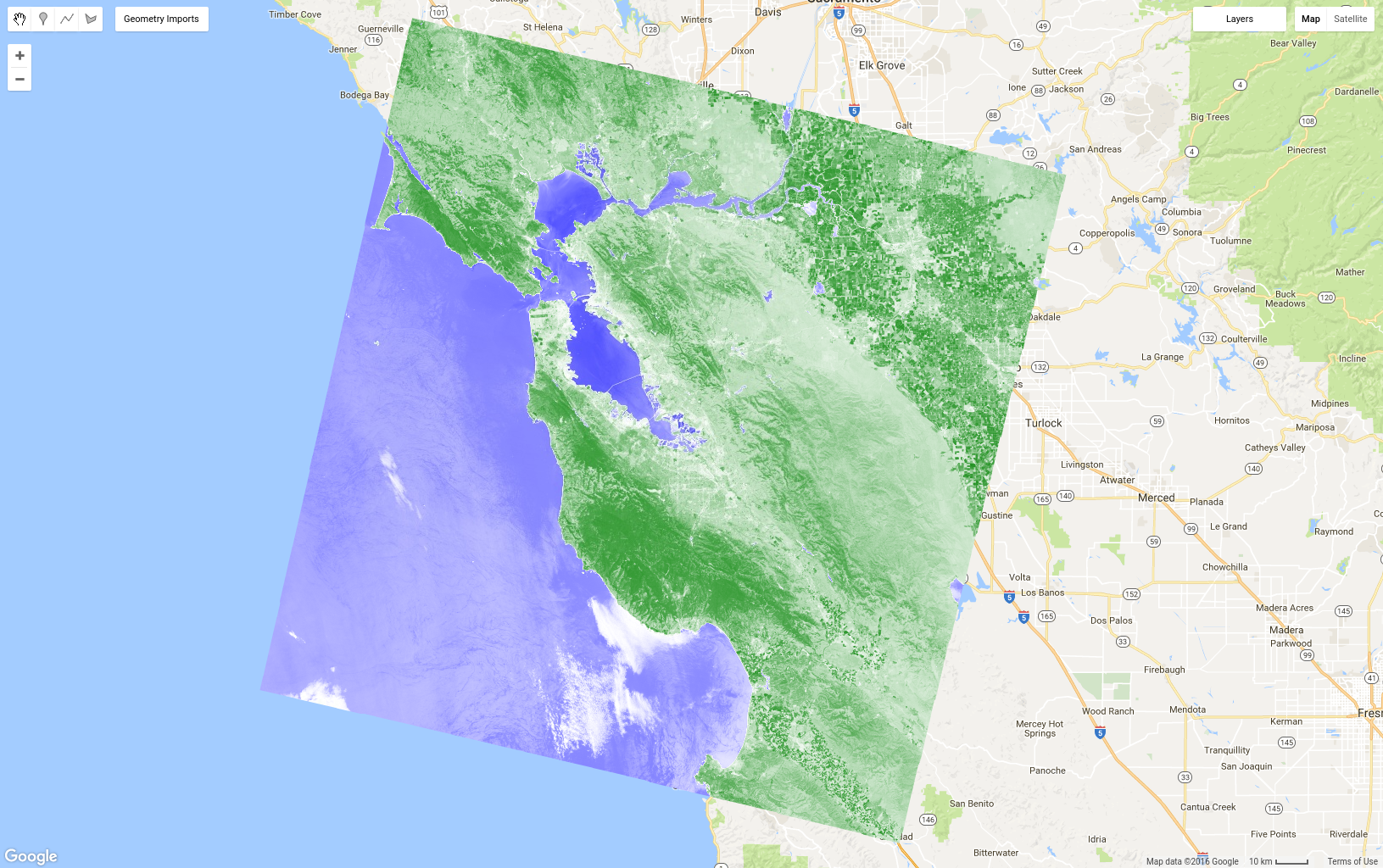# NDVI, Mapping a Function over a Collection, Quality Mosaicking

Previously, you learned how to get individual Landsat scenes by doing something like this, where `l8` and `point` are imports representing the Landsat 8 TOA collection and an area-of-interest geometry:

```// Get the least cloudy image in 2015.
var image = ee.Image(
l8.filterBounds(point)
.filterDate('2015-01-01', '2015-12-31')
.sort('CLOUD_COVER')
.first()
);
```

Suppose now that you want to compute a Normalized Difference Vegetation Index (NDVI) image from the Landsat image. Vegetation reflects light in the near-infrared (NIR) part of the electromagnetic spectrum and absorbs light in the red part (Learn more about NIR reflectance from vegetation). NDVI uses this to create a single value roughly reflecting the photosynthetic activity occurring at a pixel. The calculation is (NIR - red) / (NIR + red). This results in a number between 1 and -1, where pixels with high photosynthetic activity have a high NDVI. This is one way to compute NDVI in Earth Engine:

```// Compute the Normalized Difference Vegetation Index (NDVI).
var nir = image.select('B5');
var red = image.select('B4');

// Display the result.
Map.centerObject(image, 9);
var ndviParams = {min: -1, max: 1, palette: ['blue', 'white', 'green']};
```

The result should look something like Figure 8. Note that we use the `select()` function you learned about in the previous section on masking to get the NIR and red bands, then compute NDVI using image mathematical operators that that you have also seen before in the section on `Image` math. Finally, display the image with a palette. Here we used color names instead of hex strings in the palette. (See this external reference about CSS color for details.)Figure 8. NDVI for a single Landsat scene. Blue is low and green is high NDVI.

The normalized difference operation is so ubiquitous in remote sensing, there is a shortcut function on an `ee.Image` that is useful for simplifying the code in the previous example:

```var ndvi = image.normalizedDifference(['B5', 'B4']).rename('NDVI');
```

## Mapping a Function over a Collection

Suppose now that you want to add NDVI to every image in an image collection. The way to do that in Earth Engine is to `map()` a function over the collection. Don't confuse `map()` with the `Map` object. The former is a method on a collection, and uses map in the parallel computing sense of applying a function to every element in a collection. The function defines the operations that will be applied to every element in the collection. You have seen a simple function in the JavaScript tutorial, but now we're going to make a function that includes Earth Engine functionality. For example, copy the previous NDVI code into a function which returns the input image with an NDVI band:

```var addNDVI = function(image) {
var ndvi = image.normalizedDifference(['B5', 'B4']).rename('NDVI');
};

// Test the addNDVI function on a single image.
```

This code might not be as efficient for computing NDVI for a single image, but this function can be used as an argument to `map()` in order to add an NDVI band to every image in the collection. It's often useful to first test a function on a single image, to make sure the function is behaving as you expect. Once you've tested the function on an individual image and have determined that it does what you want, you can map it over the collection:

```var withNDVI = l8.map(addNDVI);
```

To verify that this is indeed putting an NDVI band in every image in this collection, you can add the `withNDVI` collection to the map and query a random location with the Inspector tab. You should notice that each image in the collection now has a band called `NDVI`.

## Make a greenest pixel composite

Now that you've made an image collection in which each image has an NDVI band, we can explore a new way to make composites: `qualityMosaic()`. You may have noticed discontinuities between Landsat paths, even in the median pixel composite. Part of the reason for that may be due to differences in phenology as a result of images in adjacent paths being collected at different times (specifically, 8 days apart). One way to minimize this is to try to set pixel values in the composite from roughly the same phenological stage, for example the time of maximum greenness of plants (when the leaves are on and photosynthetically active). If we let max greenness be defined by the maximum NDVI, we can use `qualityMosaic()` to make a composite in which each pixel contains the maximum NDVI pixel from the collection. Now you can make use of the added NDVI band in your `withNDVI` collection:

```// Make a "greenest" pixel composite.
var greenest = withNDVI.qualityMosaic('NDVI');

// Display the result.
var visParams = {bands: ['B4', 'B3', 'B2'], max: 0.3};
The result of this code should look something like Figure 9. Comparing Figure 9 to the median composite shown in Figure 6, observe that the greenest pixel composite is indeed much greener. However, close examination of water bodies should make a different problem apparent. Specifically, water bodies now appear cloudy. This is due to the way the `qualityMosaic()` method works: at each location, the entire time series is examined and the pixel with the maximum value in the NDVI band is set as the composite value. Because NDVI is higher over clouds than water, water areas get cloudy pixels, while vegetated areas all appear green because NDVI is highest when the vegetation in the pixel is photosynthetically active.